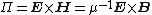# Poynting's vector

## Poynting's vector

A vector, the outward normal component of which, when integrated over a closed surface in an electromagnetic field, represents the outward flow of energy through that surface. It is given by the equation below, whereE is the electric field strength, H the magnetic field strength, B the magnetic flux density, and μ the permeability.

When an electromagnetic wave is incident on a conducting or absorbing surface, theory predicts that it should exert a force on the surface in the direction of the difference between the incident and the reflected Poynting's vector. See Electric field, Electromagnetic radiation, Maxwell's equations, Radiation pressure

McGraw-Hill Concise Encyclopedia of Physics. © 2002 by The McGraw-Hill Companies, Inc.
References in periodicals archive ?
This seems plausible since the current decay means that the corresponding longitudinal Poynting's vector then carries less power or energy with increasing distance .
where [mathematical expression not reproducible] is the complex Poynting's vector for the perturbed propagating mode, with [??] and [??] the perturbed fields calculated from (10).
The results are obtained on the bases of the energy flow of Poynting's vector through the closed surface.
The magnitude of the Poynting's vector on the earth's surface S(r, 0) = [E.sub.r](r, 0)H(r, 0), for r > a may be calculated by considering the simple formula of the magnetic field intensity given by the Ampere's law H(r, 0) = i/ (2[pi]r).
With this discrete wedge product, the discrete electric energy density E x D, magnetic energy density H x B, and Poynting's vector E x H can all be constructed.
valid for any value of the corresponding Poynting's vector [mathematical expression not reproducible].
where [EPSILON] is the surface surrounding V, [N.sub.[EPSILON]] is the outward normal to [EPSILON], [P'.sub.n] = [E'.sub.[epsilon]] x [H'.sub.n] is the Poynting's vector, V = S[delta]z.

Site: Follow: Share:
Open / Close